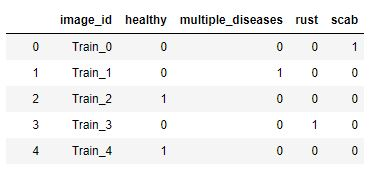# ValueError: Expected input batch_size (4) to match target batch_size (16)

Hello,

I have created a Class for the Dataset, (Code Below)

```````Preformatted text`class CustomDataset(Dataset):
def __init__(self, csv_file, id_col, target_col, root_dir, sufix=None, transform=None):
"""
Args:
csv_file   (string):             Path to the csv file with annotations.
root_dir   (string):             Directory with all the images.
id_col     (string):             csv id column name.
target_col (string):             csv target column name.
sufix      (string, optional):   Optional sufix for samples.
transform  (callable, optional): Optional transform to be applied on a sample.
"""
self.id        = id_col
self.target    = target_col
self.root      = root_dir
self.sufix     = sufix
self.transform = transform

def __len__(self):
return len(self.data)

def __getitem__(self, idx):
# get the image name at the different idx
img_name = self.data.loc[idx, self.id]

# if there is not sufic, nothing happened. in this case sufix is '.jpg'
if self.sufix is not None:
img_name = img_name + self.sufix

# it opens the image of the img_name at the specific idx
image = Image.open(os.path.join(self.root, img_name))

# if there is not transform nothing happens, here we defined below two transforms for train and for test
if self.transform is not None:
image = self.transform(image)

# define the label based on the idx
label = pd.read_csv(csv_file).loc[idx, ['healthy', 'multiple_diseases', 'rust', 'scab']].values
label = torch.from_numpy(label.astype(np.int8))
#label = label.unsqueeze(-1)

return image, label
``````

It returns a label shape: torch.size()

and then

``````train_dataset = CustomDataset(csv_file=data_dir+'train.csv', root_dir=data_dir+'images', **params)

``````

But I have this message Error:" ValueError: Expected input batch_size (4) to match target batch_size (16)."

``````for idx, (data, target) in enumerate(loaders):

## find the loss and update the model parameters accordingly
## record the average training loss, using something like
## train_loss = train_loss + ((1 / (batch_idx + 1)) * (loss.data - train_loss))
# forward pass: compute predicted outputs by passing inputs to the model
#print(data)
output = model(data)
# calculate the batch loss
#torch.max(target, 1)
print('output shape:  ', output.shape)
#target = target.view(-1)
print('target shape:  ', target.shape)

loss = criterion(output, target)
``````

I can see that the

• Output shape is: torch.size([4, 133])
• Target shape is: torch.size([4, 4])

I know that my target should be () and as the label shape of the dataset is this shape I don’t understand why it changed to [(4, 4)]).

I don’t understand what I missed and how can I get the target shape to be ()

I tried to insert in the back propagation the below, basically the idea was to replace (in bold) the idx (data, target) that is dimension ([4, 4]) with a label_inter, which is the same that is used in the dataset.

``````label_inter = pd.read_csv(data_dir+'train.csv').iloc[idx, 1:5].value
label_inter = torch.from_numpy(label_inter.astype(np.int64))
label_inter = label_inter.squeeze(-1)
label_inter = label_inter.view(-1)
``````

But then I have an error

ValueError: Expected input batch_size (1) to match target batch_size (4)

after the Idx 455, but I checked and there is nothing specific on the picture Train_456.jpg

So this doesn’t work neither. Any suggestions? Why the dataloader change the dimension of my label?

Note the picture are categorized as below:@Arnaud_Mal how did you load the data. I have exact same data, but I am not able to load it.

Hi @aleemsidra,

Are you in local or in Kaggle jupyter notebook?

I am on my local machine.